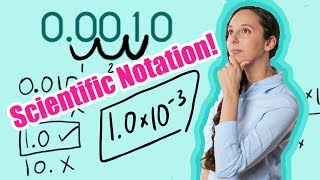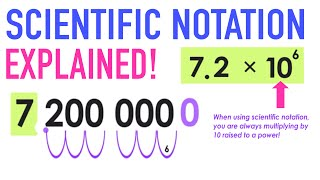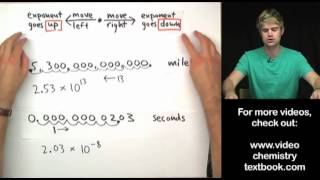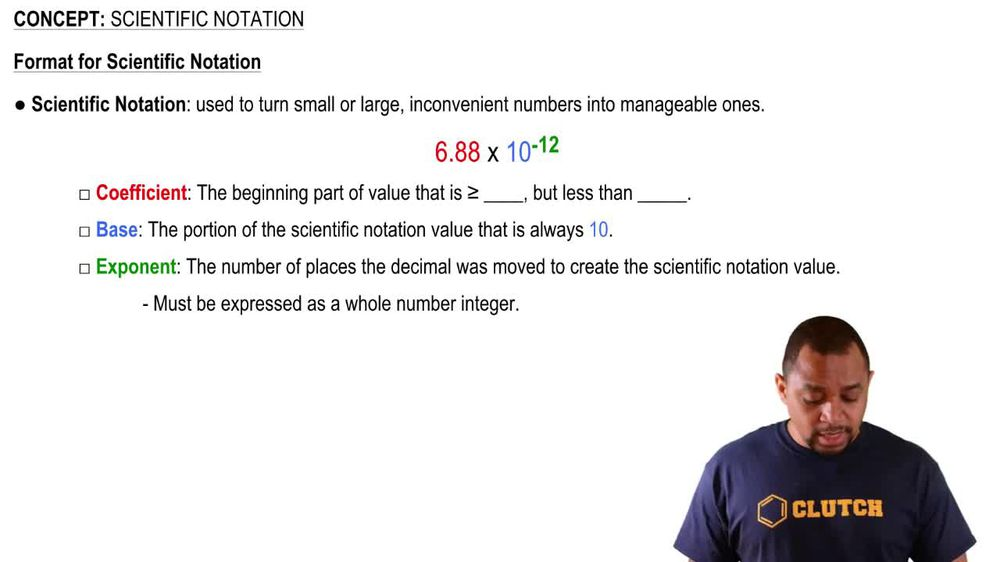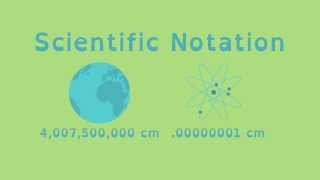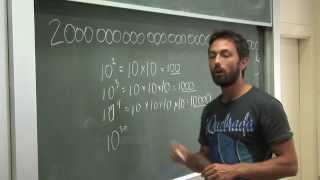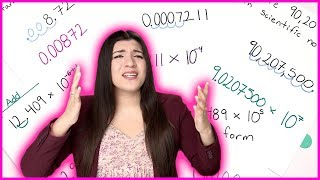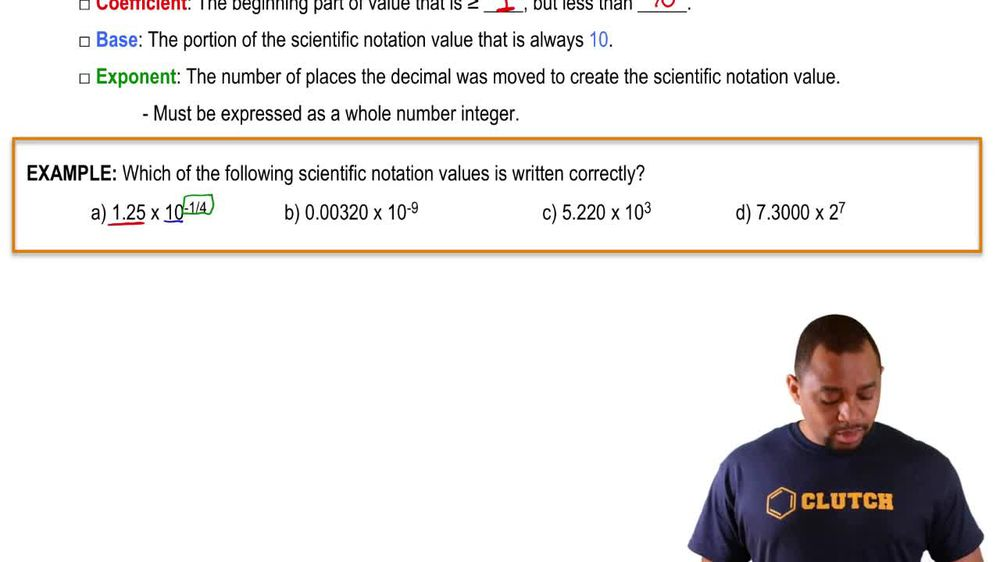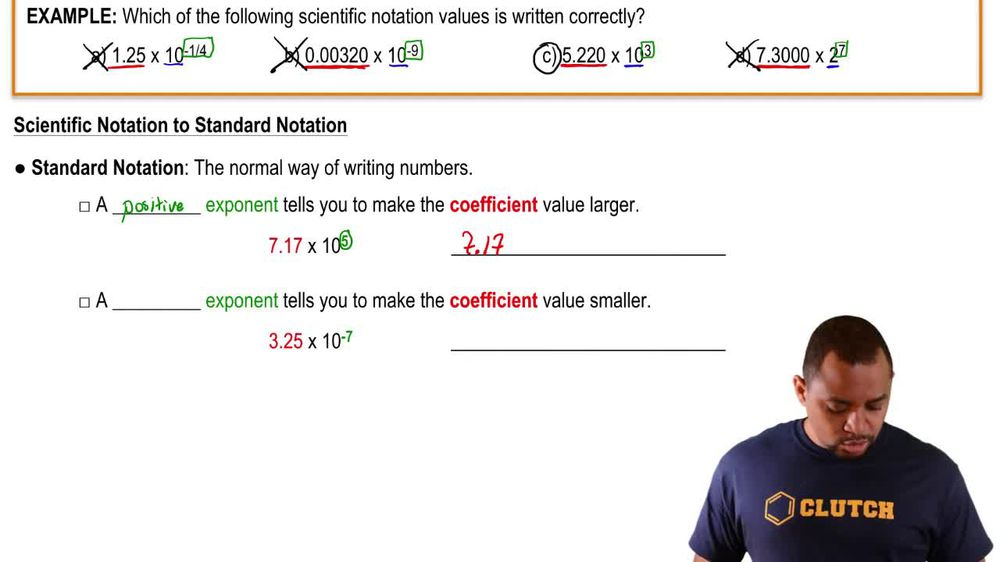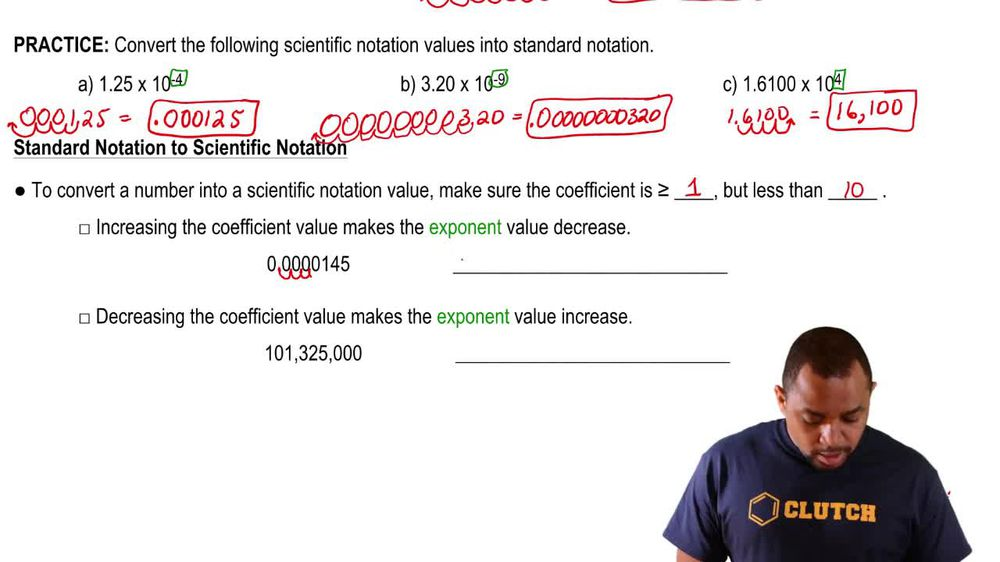Start typing, then use the up and down arrows to select an option from the list.
1. 1. Intro to General Chemistry2. Scientific Notation
Problem

# Express the following measurements in scientific notation. (b) 0.000 042 1 mL

Relevant Solution1m
Play a video:
Hey, welcome back. Alright, so the circumference of Earth is 40,780 km. Right? So that's our number. We want to express this number in the standard exponential notation or scientific notation is the same thing. After rounding to two significant figures. Alright, so looking at this number right now obviously has more than two significant figures. Right, But we want to. So the two significant figures that we have there are significant R. Four and zero. Right? But next to zero we have seven. Right? So which means that we actually have to round up the zero to a one. Okay, so it's not 40 but it's 41. Now, scientific notation is usually from 1 to 10, so this is going to be 4.1 and then we do need to add the exponents here. So it's going to be 10 times to what power. So if we take a look here we are moving 12344 decimal places. We're making this larger number into smaller number. So this is going to be times 10 to the positive four. Okay, that is going to be our answer with two significant figures and that is option C. Okay, 4.1 Times 10 to the positive for thanks so much for watching